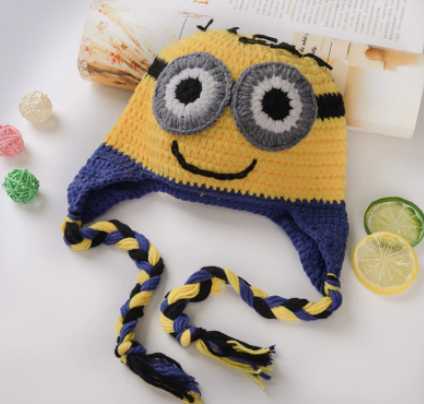Home
06 February 2021 ( 151 views )# Making a Minion-Shaped Hat for 3 Years Old

Hello!

Today we brought you a very cute hat recipe. Kids will love these fun hats.

So let's start knitting right now!

Materials
Yellow Knitting Yarn
Blue Hand Knitting Yarn
Black Knitting Yarn
White Hand Knitting Yarn
Gray Knitting Yarn
2.5 mm skewer
2 mm crochet hook
Abbreviations
Sh: magic ring

X: tight needle

V: increase

Dc: handrail

Dcv: increase handrailFabrication

1. Let's put on 5 loops.

2-3. Let's knit 5 loops straight.

4. Let's increase the edges one by one. (7)

5. Let's knit 7 loops straight.

6. Let's increase one loop from the edges. (9)

7. Let's knit 9 loops straight.

8. Let's increase the sides one by one. (11th)

9. Let's knit 11 loops straight.

10. Let's increase one by one. (13)

11. Let's knit 13 loops straight.

13. Let's increase the edges one by one. (15)

14. Let's knit 15 stitches straight.

15. Let's increase one by one. (17)

16. Let's knit 17 loops straight.

17. Let's increase the edges one by one. (19)

18-20. Let's knit 19 loops straight.

21. Let's knit one loop straight from the edges. (21)

22-24. Let's knit 21 stitches straight.

25. Let's increase one by one. (23)

26-28. Let's knit 23 loops straight.